# Fibonacci Sequence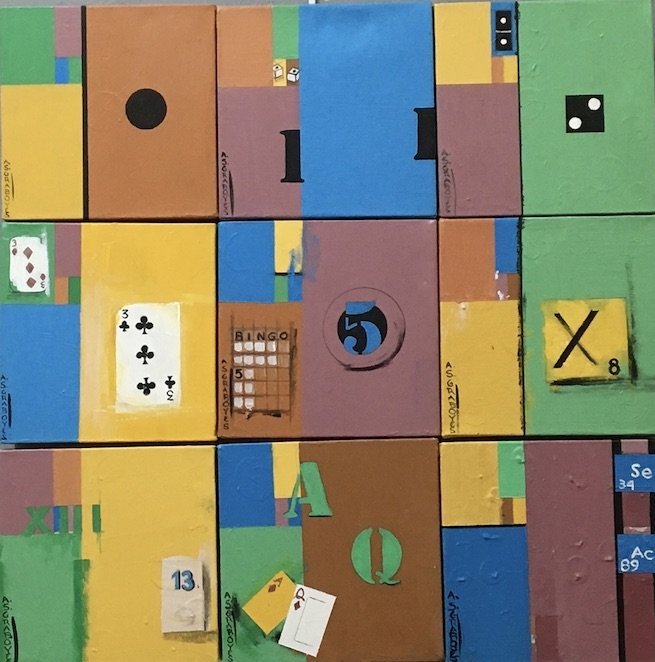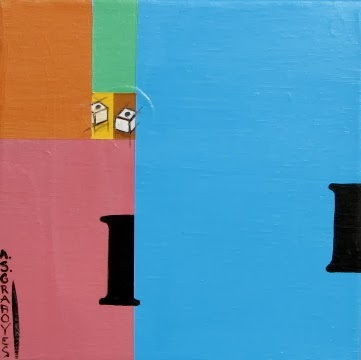Fibonacci 1…1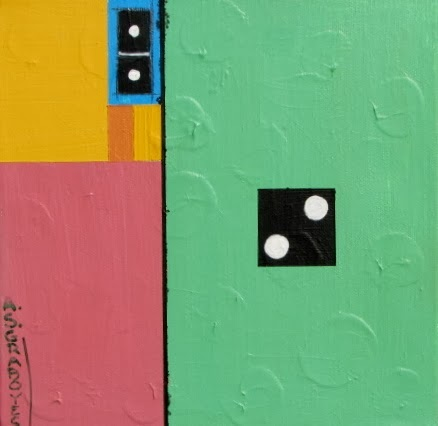Fibonacci 2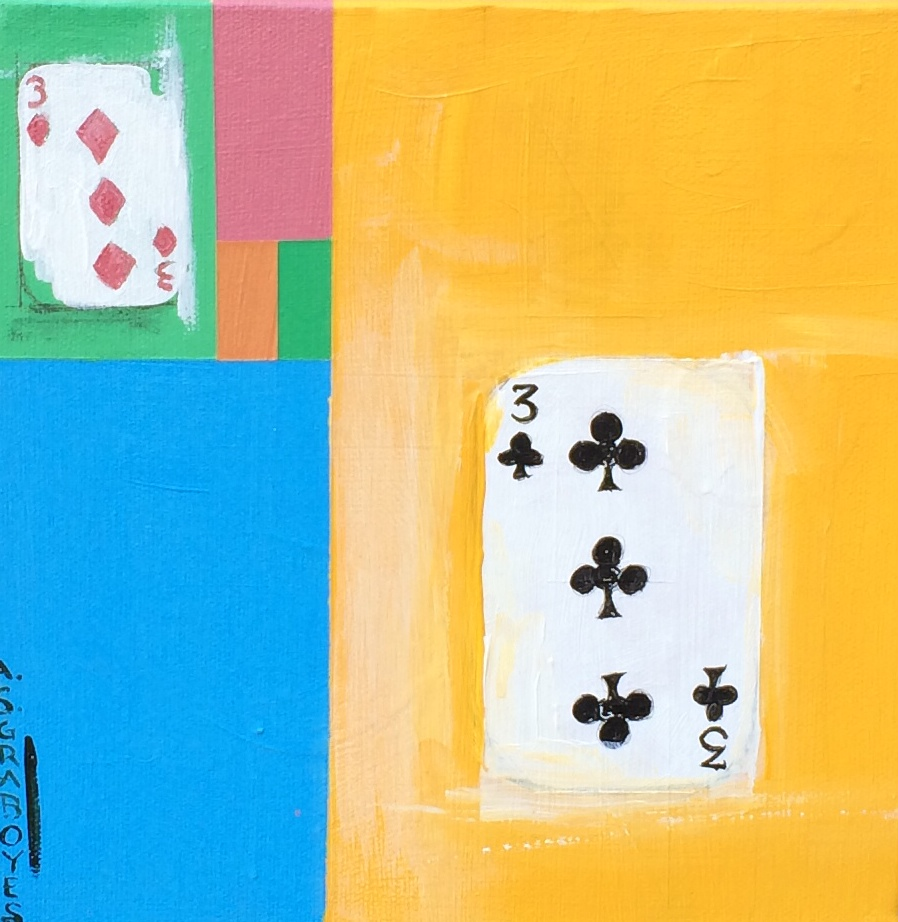Fibonacci 3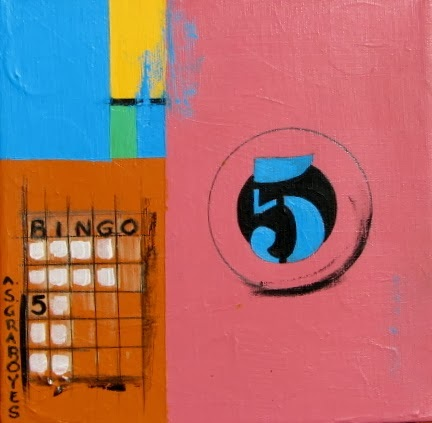Fibonacci 5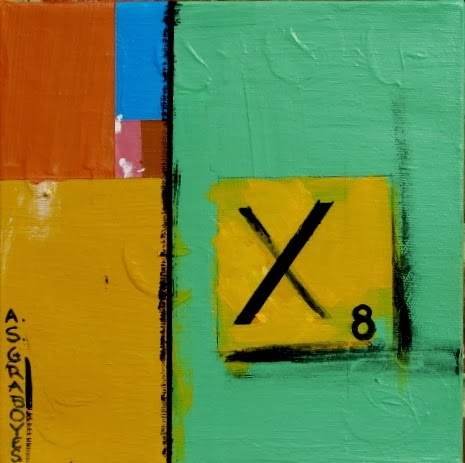Fibonacci 8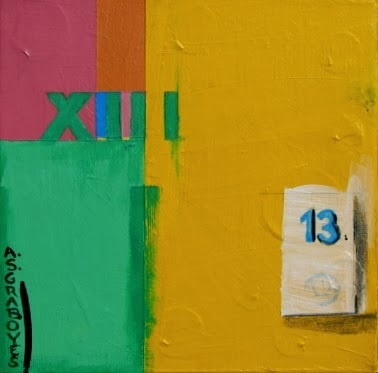Fibonacci 13Fibonacci 21Fibonacci 34, 55, 89

Fibonacci Sequence
each painting is 8″x8″ on gallery wrapped canvas and all nine sold as a set

\$465 + tax, shipping

The Fibonacci sequence has captivated mathematicians, artists, designers, and scientists for centuries. Also known as the Golden Ratio and appears frequently in nature: flower petals, seed heads, pinecones, fruits & vegetables, tree branches, shells, spiral galaxies.
The Fibonacci sequence starts like this: 0, 1, 1, 2, 3, 5, 8, 13, 21, 34, 55 and so on forever. Each number is the sum of the two numbers that precede it.Author: asgraboyes